## 2.14Diﬀusion

The momentum equation for a homogeneous, incompressible, Newtonian ﬂuid is presented in Eq. (2.47 ). In the case of zero body force,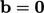, and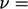constant, Eq. (2.47 ) becomes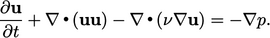(2.49)
This is a typical transport equation characterised by:
• the local rate of change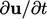, described in Sec. 2.5 ;
• advection of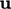by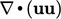, described in Sec. 2.8 ;
• diﬀusion of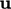by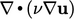;
• a “source” due to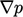, described in Sec. 2.12 .

Note that, since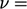constant,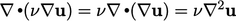, where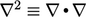denotes the Laplace operator.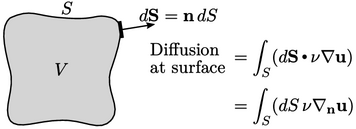The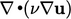term is a special form of divergence in which the ﬂux includes the surface normal gradient denoted by the operator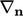where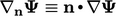.

The term models diﬀusion across the surface of the ﬂuid element. Diﬀusion generally represents the transport of a ﬂuid property — here, momentum — due to ﬂuctuating motions that are not captured by the bulk motion that is represented by the continuum velocity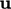.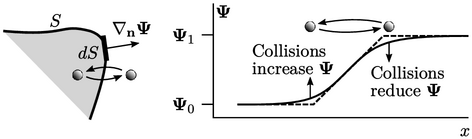Fluctuations include any random motion of particle constituents of matter, e.g. molecules, and turbulent structures. Through these motions, particles can pass across a surface boundary, transporting property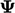through a gradient of(above, left).

Particles carrying highermove into regions of particles with lowerand vice versa. Through particle collisions, high values oftend to reduce and low values increase (right).

### Laplacian

“Laplacian”11 describes a term of the form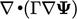where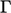is a diﬀusivity coeﬃcient.12 A Laplacian term is conservative since all variables are to the right of a divergence, as described in Sec. 2.9 . It is also bounded since it tends to decrease high values and increase low values as shown above.

The Laplacian represents a ﬂux due to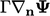across the surface, per unit volume, as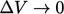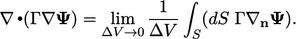(2.50)

11After Pierre-Simon Laplace, Théorie des attractions des sphéroïdes et de la ﬁgure des planètes, 1785 but described in 1761 both by Leonhard Euler and Jean-Baptiste le Rond d’Alembert.
12More precisely, “Laplacian” denotes a term without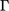, i.e.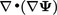, represented as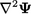but we includehere because it is generally needed.

Notes on CFD: General Principles - 2.14 Diﬀusion